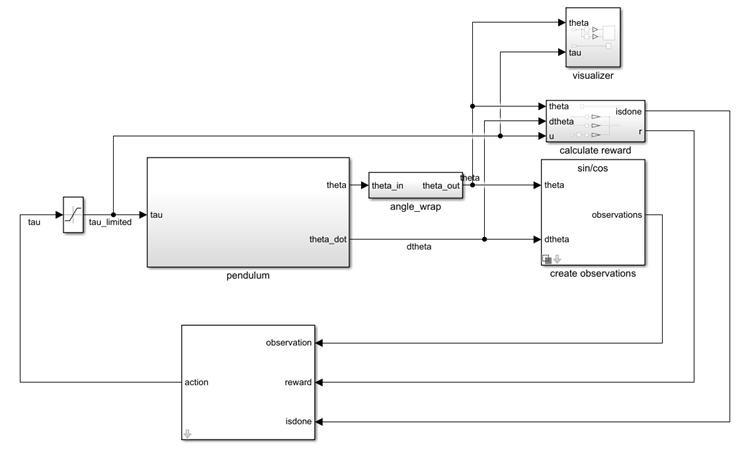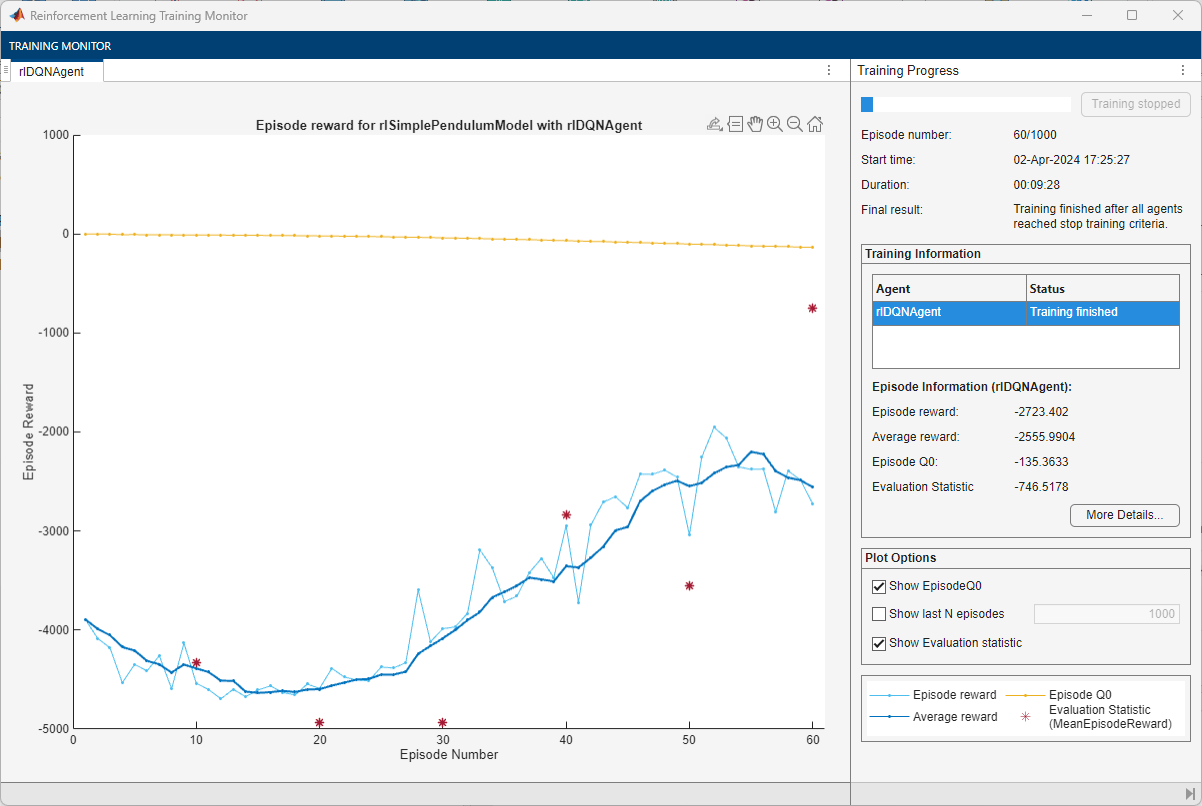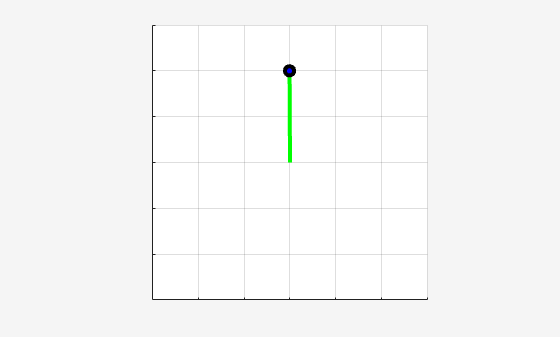# Train DQN Agent to Swing Up and Balance Pendulum

This example shows how to train a deep Q-learning network (DQN) agent to swing up and balance a pendulum modeled in Simulink®.

For more information on DQN agents, see Deep Q-Network (DQN) Agents. For an example that trains a DQN agent in MATLAB®, see Train DQN Agent to Balance Cart-Pole System.

### Pendulum Swing-Up Model

The reinforcement learning environment for this example is a simple frictionless pendulum that initially hangs in a downward position. The training goal is to make the pendulum stand upright without falling over using minimal control effort.

Open the model.

```mdl = "rlSimplePendulumModel"; open_system(mdl)```For this model:

• The upward balanced pendulum position is 0 radians, and the downward hanging position is `pi` radians.

• The torque action signal from the agent to the environment is from –2 to 2 N·m.

• The observations from the environment are the sine of the pendulum angle, the cosine of the pendulum angle, and the pendulum angle derivative.

• The reward ${\mathit{r}}_{\mathit{t}}$, provided at every timestep, is

`${\mathit{r}}_{\mathit{t}}=-\left({{\theta }_{\mathit{t}}}^{2}+0.1{\stackrel{˙}{{\theta }_{\mathit{t}}}}^{2}+0.001{\mathit{u}}_{\mathit{t}-1}^{2}\right)$`

Here:

• ${\theta }_{\mathit{t}}$ is the angle of displacement from the upright position.

• $\stackrel{˙}{{\theta }_{\mathit{t}}}$ is the derivative of the displacement angle.

• ${\mathit{u}}_{\mathit{t}-1}$ is the control effort from the previous time step.

### Create Environment Interface

Create a predefined environment interface for the pendulum.

`env = rlPredefinedEnv("SimplePendulumModel-Discrete")`
```env = SimulinkEnvWithAgent with properties: Model : rlSimplePendulumModel AgentBlock : rlSimplePendulumModel/RL Agent ResetFcn : [] UseFastRestart : on ```

The interface has a discrete action space where the agent can apply one of three possible torque values to the pendulum: –2, 0, or 2 N·m.

To define the initial condition of the pendulum as hanging downward, specify an environment reset function using an anonymous function handle. This reset function sets the model workspace variable `theta0` to `pi`.

`env.ResetFcn = @(in)setVariable(in,"theta0",pi,"Workspace",mdl);`

Get the observation and action specification information from the environment.

`obsInfo = getObservationInfo(env)`
```obsInfo = rlNumericSpec with properties: LowerLimit: -Inf UpperLimit: Inf Name: "observations" Description: [0x0 string] Dimension: [3 1] DataType: "double" ```
`actInfo = getActionInfo(env)`
```actInfo = rlFiniteSetSpec with properties: Elements: [3x1 double] Name: "torque" Description: [0x0 string] Dimension: [1 1] DataType: "double" ```

Specify the simulation time `Tf` and the agent sample time `Ts` in seconds.

```Ts = 0.05; Tf = 20;```

Fix the random generator seed for reproducibility.

`rng(0)`

### Create DQN Agent

A DQN agent approximates the long-term reward, given observations and actions, using a parametrized Q-value function critic.

Since DQN agents have a discrete action space, you have the option to create a vector (that is a multi-output) Q-value function critic, which is generally more efficient than a comparable single-output critic. A vector Q-value function is a mapping from an environment observation to a vector in which each element represents the expected discounted cumulative long-term reward when an agent starts from the state corresponding to the given observation and executes the action corresponding to the element number (and follows a given policy afterwards).

To model the Q-value function within the critic, use a deep neural network. The network must have one input layer (which receives the content of the observation channel, as specified by `obsInfo`) and one output layer (which returns the vector of values for all the possible actions). Note that `prod(obsInfo.Dimension)` returns the number of dimensions of the observation space (regardless of whether they are arranged as a row vector, column vector, or matrix, while `numel(actInfo.Elements)` returns the number of elements of the discrete action space.

Define the network as an array of layer objects.

```criticNet = [ featureInputLayer(prod(obsInfo.Dimension)) fullyConnectedLayer(24) reluLayer fullyConnectedLayer(48) reluLayer fullyConnectedLayer(numel(actInfo.Elements)) ];```

Convert to `dlnetwork` and display the number of weights.

```criticNet = dlnetwork(criticNet); summary(criticNet)```
``` Initialized: true Number of learnables: 1.4k Inputs: 1 'input' 3 features ```

View the critic network configuration.

`plot(criticNet)`For more information on creating value functions that use a deep neural network model, see Create Policies and Value Functions.

Create the critic using `criticNet`, as well as observation and action specifications. For more information, see `rlVectorQValueFunction`.

`critic = rlVectorQValueFunction(criticNet,obsInfo,actInfo);`

Specify options for the critic optimizer using `rlOptimizerOptions`.

`criticOpts = rlOptimizerOptions(LearnRate=0.001,GradientThreshold=1);`

To create the DQN agent, first specify the DQN agent options using `rlDQNAgentOptions`.

```agentOptions = rlDQNAgentOptions(... SampleTime=Ts,... CriticOptimizerOptions=criticOpts,... ExperienceBufferLength=3000,... UseDoubleDQN=false);```

Then, create the DQN agent using the specified critic and agent options. For more information, see `rlDQNAgent`.

`agent = rlDQNAgent(critic,agentOptions);`

### Train Agent

To train the agent, first specify the training options. For this example, use the following options.

• Run each training for at most 1000 episodes, with each episode lasting at most 500 time steps.

• Display the training progress in the Episode Manager dialog box (set the `Plots` option) and disable the command line display (set the `Verbose` option to `false`).

• Stop training when the agent receives an average cumulative reward greater than –1100 over five consecutive episodes. At this point, the agent can quickly balance the pendulum in the upright position using minimal control effort.

• Save a copy of the agent for each episode where the cumulative reward is greater than –1100.

For more information, see `rlTrainingOptions`.

```trainingOptions = rlTrainingOptions(... MaxEpisodes=1000,... MaxStepsPerEpisode=500,... ScoreAveragingWindowLength=5,... Verbose=false,... Plots="training-progress",... StopTrainingCriteria="AverageReward",... StopTrainingValue=-1100,... SaveAgentCriteria="EpisodeReward",... SaveAgentValue=-1100);```

Train the agent using the `train` function. Training this agent is a computationally intensive process that takes several minutes to complete. To save time while running this example, load a pretrained agent by setting `doTraining` to `false`. To train the agent yourself, set `doTraining` to `true`.

```doTraining = false; if doTraining % Train the agent. trainingStats = train(agent,env,trainingOptions); else % Load the pretrained agent for the example. load("SimulinkPendulumDQNMulti.mat","agent"); end```### Simulate DQN Agent

To validate the performance of the trained agent, simulate it within the pendulum environment. For more information on agent simulation, see `rlSimulationOptions` and `sim`.

```simOptions = rlSimulationOptions(MaxSteps=500); experience = sim(env,agent,simOptions);```